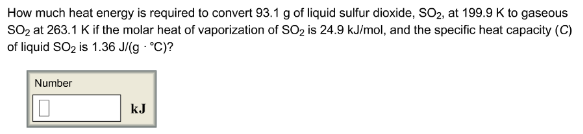# Problem: How much heat energy is required to convert 93.1 g of liquid sulfur dioxide, SO2, at 199.9 K to gaseous SO2 at 263.1 K if the molar heat of vaporization of SO2 is 24.9 kJ/mol, and the specific heat capacity (C) of liquid SO2 is 1.36 J/(g·°C)?

###### FREE Expert Solution
90% (121 ratings)###### Problem Details

How much heat energy is required to convert 93.1 g of liquid sulfur dioxide, SO2, at 199.9 K to gaseous SO2 at 263.1 K if the molar heat of vaporization of SO2 is 24.9 kJ/mol, and the specific heat capacity (C) of liquid SO2 is 1.36 J/(g·°C)?